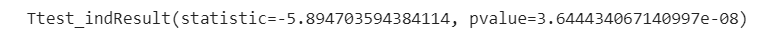# Statistics with python, Part 1Photo by Christopher Burns on Unsplash

## Easy python, no installing

Let’s use Google Colaboratory to write python code!

`viewer = "😎 Physicist" ## variable assingmentprint(f"Hello world {viewer}")`
`normal_dist = np.random.normal(30,5,60)`
`import matplotlib.pyplot as pltfigure = plt.hist(normal_dist)`
`normal_dist2 = np.random.normal(34,3,60)`
`fig1 = plt.hist(normal_dist)fig2 = plt.hist(normal_dist2)`
`from scipy.stats import ttest_indtest = ttest_ind(normal_dist,normal_dist2)print(test)`Result, the statistic is -5, and p-value = 1e-8
`from scipy.stats import ttest_reltest = ttest_rel(normal_dist,normal_dist2)print(test)`
`from scipy.stats import wilcoxontesttest = wilcoxon(normal_dist,normal_dist2)print(test)`
`from scipy.stats import mannwhitneyutest = mannwhitneyu(normal_dist,normal_dist2)print(test)`

--

--